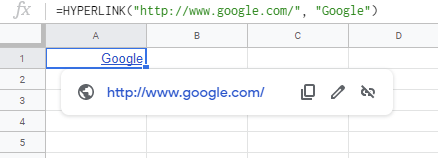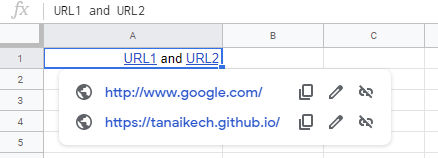Gists

Recently, it seems that the specification of Google Spreadsheet was updated. Before this, when a cell has only one hyperlink. In this case, the hyperlink was given to a cell using =HYPERLINK("http://www.google.com/", "Google") as following figure.But by the recent update, a cell got to be able to have multiple hyperlinks as following figure. In this case, the hyperlinks are set by the RichTextValue object.In this report, I would like to introduce the method for setting and retrieving the multiple URLs for a cell.

## Set multiple URLs to a cell

In this section, I would like to introduce the method for setting the multiple URLs to a cell using the sample script. As the sample situation, above figure is achieved using a sample script.

### Sample script

const sheet = SpreadsheetApp.getActiveSpreadsheet().getSheetByName("Sheet1");
.setText("url1 and url2")
.build();
sheet.getRange("A1").setRichTextValue(RichTextValue);

• In this script, at first, the RichTextValue object is created. Then, it is put to a cell.

## Get multiple URLs from a cell

In this section, I would like to introduce the method for retrieving the multiple URLs from a cell using the sample script. As the sample situation, the URLs are retrieved from above figure.

### Sample script

const sheet = SpreadsheetApp.getActiveSpreadsheet().getSheetByName("Sheet1");
const range = sheet.getRange("A1");
const RichTextValue = range.getRichTextValue().getRuns();
const res = RichTextValue.reduce((ar, e) => {
if (url) ar.push(url);
return ar;
}, []);
console.log(res);


## Note

• In this case, when =HYPERLINK("http://www.google.com/", "Google") is put to a cell, the URL can be retrieved using above script. Of course, you can also retrieve this using getFormula.

• When all text in a cell has an URL, you can also retrieved the URL from the cell using the following simple script.

const sheet = SpreadsheetApp.getActiveSpreadsheet().getSheetByName("Sheet1");

• In the current stage (May 15, 2020), at the official document, there are no methods of setLinkUrl and getLinkUrl, in Class RichTextValueBuilder and Class RichTextValue. But I believe that those will be added in the near future update.